Version: 2012-12-04 21:25:33

I'm finding slight differences between the 95% profile confidence intervals generated by R and ADMB, although the profiles themselves match pretty closely. This is my attempt to write up a reproducible example. I was initially making a stupid mistake (confusing likelihoods returned by ADMB with log-likelihoods!), but I am now reasonably convinced that the problem is that ADMB computes confidence intervals by integrating the scaled likelihood to find the position of the $$\alpha/2$$% tails, rather than using the standard “cutoff” approach. If the profile is wonky at the edges of the profiled region, that will make the confidence intervals wonky too.

## Preliminaries

Data files, TPL files, and the vfit package, are available at http://www.math.mcmaster.ca/bolker/misc/admb_prof.

library(R2admb)  ## R to ADMB interface
library(vfit)  ## R code for generating data, fitting models etc,
## data set 2:
set.seed(1005)
dvar1 <- diff(apply(simgrowth(g0 = 3, s0 = 4, nind = 200)$size, 2, var)) ## example from a real data set (Callidryas Block=4, Tank=5) dvar2 <- c(0.00965825930227891, 0.0409751281929446, 0.218256211403151, 0.154333281909493, 0.792065497615383)  fn <- "deltavar_prof" compile_admb(fn)  ## Data set 1 write_pin(fn, list(rho = 0.5, var_g = 1)) write_dat(fn, list(nt = length(dvar1) + 1, dvar = dvar1, max_var_g = 200)) ## save a copy of this data file file.copy("deltavar_prof.dat", "deltavar_prof1.dat", overwrite = TRUE) run_admb(fn, profile = TRUE)  ## Warning: running command './deltavar_prof -lprof > deltavar_prof.out' had ## status 1  Not quite sure why I'm getting status 1 here, but it only happens if I run with profiling enabled (the command line is ./deltavar_prof -lprof). Is ADMB trying to tell me that something's wrong (e.g. are we hitting the max var boundary for some values of rho, or are we getting convergence failures in part of the profiling), or is there just something funny about the return values in this case? r1 <- read_admb(fn, profile = TRUE)  Extract profile information and scale it (convert to relative deviance) read_admb gets this stuff from the prof_rho.plt file p1A <- r1$prof$prof_rho pp1A <- as.data.frame(p1A$prof)
pp1A$dev <- -2 * log(pp1A[, 2]) pp1A$dev <- pp1A$dev - min(pp1A$dev)


Fit in R for comparison (details hidden: see the vfit package)

vR1 <- vfit(dvar = dvar1)  ## fit & profile in R
pp1R <- subset(as.data.frame(vR1$profile), param == "rho")  Brute-force profiling with ADMB: fn0 <- "deltavar_prof_fixrho" compile_admb(fn0)  write_dat(fn0, list(nt = length(dvar1) + 1, dvar = dvar1, max_var_g = 10)) rhovec <- seq(0, 1, by = 0.05) hackprof1 <- numeric(length(rhovec)) for (i in 1:length(rhovec)) { write_pin(fn0, list(rho = rhovec[i], var_g = 1)) run_admb(fn0) r3 <- read_admb(fn0) hackprof1[i] <- -2 * logLik(r3) }  Compare profiles and confidence intervals: par(las = 1, bty = "l") plot(dev ~ value, data = pp1A, type = "o", xlab = "rho", ylab = "deviance", cex = 0.5) lines(z^2 ~ par.vals.rho, data = pp1R, type = "o", col = 2, pch = 2, cex = 0.5) abline(v = vR1$ci, lty = 2, col = 2)
abline(h = 2 * qnorm(0.975), col = "gray")
abline(v = p1A$ci[2, -1], lty = 2) lines(rhovec, hackprof1 - min(hackprof1), type = "o", col = 4, pch = 3) legend("top", legend = c("R", "ADMB auto", "ADMB hack"), pch = c(2, 1, 3), col = c(2, 1, 4), bty = "n", cex = 0.8)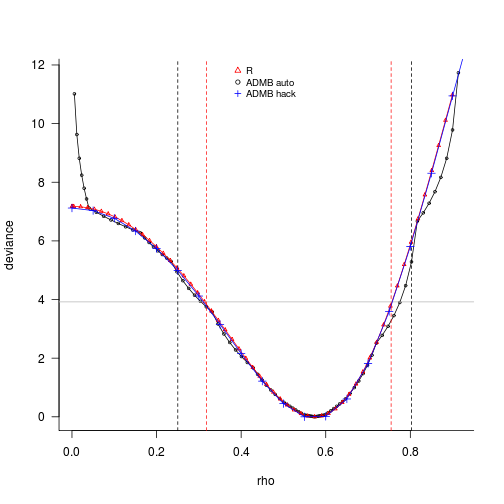We can see that the profiles match over the central range, but that things get a bit wonky near the very edges of the feasible range for rho. Not sure why: in all my checks, R and ADMB are producing the same log-likelihood surface (i.e. computed log-likelihoods agree for any particular parameter combination). I looked at the inflection points for each value of rho (cyan lines), and tried changing the starting points of the estimate to var_g=3; didn't seem to make a difference … par(bty = "l", las = 1) cc <- plotsurf(dvar1, vlim = c(2, 5), nll_levels = 0:10, a_levels = NULL) with(cc, contour(x, y, z, levels = min(z) + qchisq(0.95, 1)/2, labels = "0.95", add = TRUE, col = "blue", lty = 2)) abline(v = vR1$ci, lty = 2)
abline(v = confint(r1, "profile"), lty = 3)
vv <- subset(as.data.frame(vR1$prof), param = "rho") with(vv, lines(focal, par.vals.var_g, lty = 3)) ipos <- t(apply(cc$z, 1, function(x) {
ctr <- which.min(abs(diff(x)))
-ctr)))
}))
with(cc, matlines(x, matrix(y[ipos], ncol = 2), col = "cyan"))
with(cc, lines(x, y[ipos[, 2]]))
## fitted MLEs are identical ...
with(as.list(coef(vR1)), points(rho, var_g))
with(as.list(coef(r1)), points(rho, var_g, pch = 3))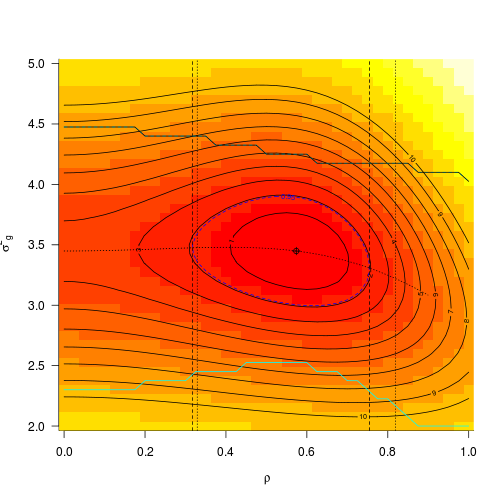Contours on this graph are labeled according to negative log-likelihood (half of deviance). As far as I can tell, the R fit is correct (???) here, and the problem with the ADMB profile confidence intervals is a combination of (1) the wonkiness of the profile toward the extremes (???) and (2) that in contrast to the typical profile likelihood interval calculation (find the value such that the conditional minimum of the deviance is equal to the minimum deviance + $$\chi^2(0.95,1)=3.84$$), ADMB is finding the confidence intervals by integrating the scaled likelihood and finding the parameter values that give the cumulative integral close to $$\{\alpha/2,1-\alpha/2\}$$ (see get_confidence_interval in src/nh99/newmodm3.cpp); this is sensitive to wonkiness in the tails.

Clean up:

clean_admb(fn, "output")


## Data set 2

This is a case (real data!) that leads to much bigger differences.

write_dat(fn, list(nt = length(dvar2) + 1, dvar = dvar2, max_var_g = 200))
file.copy("deltavar_prof.dat", "deltavar_prof2.dat", overwrite = TRUE)

## Warning: running command './deltavar_prof -lprof > deltavar_prof.out' had
## status 1

r2 <- read_admb(fn, profile = TRUE)
p2A <- r2$prof$prof_rho
pp2A <- as.data.frame(p2A$prof) pp2A$dev <- -2 * log(pp2A[, 2])  ## fix!
pp2A$dev <- pp2A$dev - min(pp2A$dev) vR2 <- vfit(dvar = dvar2) ## fit & profile in R pp2R <- subset(as.data.frame(vR2$profile), param == "rho")


The ADMB profile looks quite odd: (1) rho values greater than the upper bound (rho=1) are evaluated; (2) the estimated deviances are either tiny or huge …

summary(pp2A, digits = 3)

##      value         likelihood       dev
##  Min.   :0.999   Min.   :  0   Min.   :  0.00
##  1st Qu.:0.999   1st Qu.:  0   1st Qu.:  0.00
##  Median :1.000   Median :658   Median :  0.01
##  Mean   :1.000   Mean   :430   Mean   : 72.70
##  3rd Qu.:1.000   3rd Qu.:661   3rd Qu.:230.26
##  Max.   :1.001   Max.   :662   Max.   :230.26


Plot the profiles (left, regular rho scale: right, logit-rho scale).

op <- par(las = 1, bty = "l")
par(mfcol = c(1, 2))
plot(pmin(dev, 15) ~ value, data = pp2A, type = "o", xlab = "rho", ylab = "deviance",
cex = 0.5, xlim = c(0, 1.2), ylim = c(0, 15))
lines(z^2 ~ par.vals.rho, data = pp2R, type = "o", col = 2, pch = 2, cex = 0.5)
abline(v = vR2$ci, lty = 2, col = 2) abline(h = 2 * qnorm(0.975), col = "gray") abline(v = p2A$ci[2, -1], lty = 2)
legend("topleft", col = 1:2, pch = 1, legend = c("ADMB", "R"), bty = "n")
## logit scale
plot(dev ~ qlogis(value), data = pp2A, type = "o", xlab = "logit(rho)", ylab = "",
cex = 0.5, xlim = c(-7, 16), ylim = c(0, 15))

## Warning: NaNs produced

lines(z^2 ~ qlogis(par.vals.rho), data = pp2R, type = "o", col = 2, pch = 2,
cex = 0.5)
abline(v = vR2$ci, lty = 2, col = 2) abline(h = 2 * qnorm(0.975), col = "gray") abline(v = p2A$ci[2, -1], lty = 2)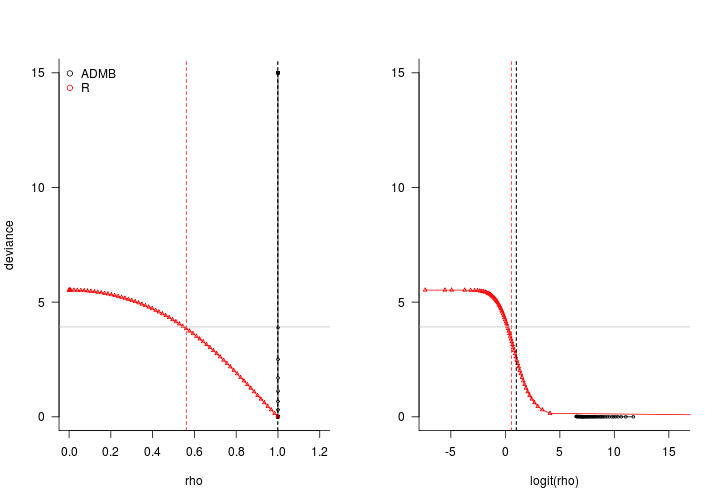par(op)


Compare Dave Fournier's results (4 Dec 2012): these results are those read in from the prof_rho.plt file he sent; the processed file with the profile extracted; and my results from above.

par(las = 1, bty = "l")
file.copy("prof_rho.plt_DF", "prof_rho_DF.plt")
with(as.data.frame(r4$prof), plot(-2 * log(likelihood) ~ value)) t2 <- read.table("t.2", header = FALSE) lines(t2[, 1], -2 * log(t2[, 2]), col = 2) with(pp1A, lines(value, -2 * log(likelihood), col = 4, lty = 2))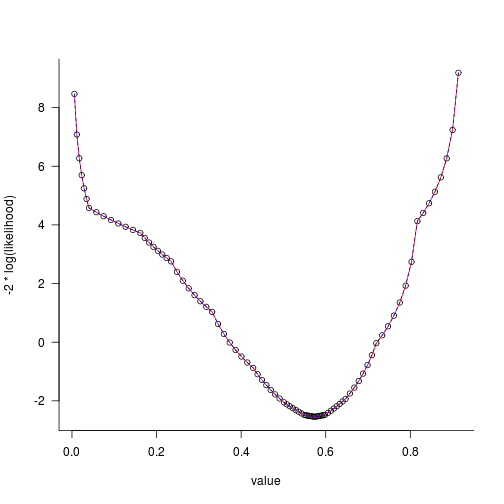The surface looks reasonable: par(bty = "l", las = 1) cc <- plotsurf(dvar2, nll_levels = 0:10, a_levels = NULL) with(cc, contour(x, y, z, levels = min(z) + qchisq(0.95, 1)/2, labels = "0.95", add = TRUE, col = "blue", lty = 2)) abline(v = vR2$ci, lty = 2)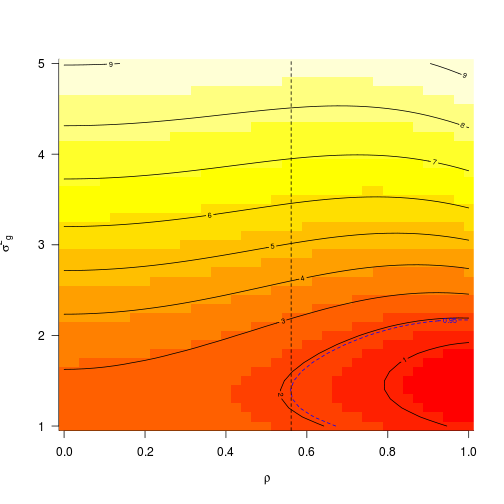We can construct a profile by hand (by fitting conditional maxima at a variety of values of rho); deltavar_prof_fixrho is just like deltavar_prof except that the phase of rho is set negative (i.e rho is a fixed variable), and we take out the definition of prof_rho as a profiling variable. We get a profile that matches R's exactly:

write_dat(fn0, list(nt = length(dvar2) + 1, dvar = dvar2, max_var_g = 10))
rhovec <- seq(0, 1, by = 0.05)
hackprof2 <- numeric(length(rhovec))
for (i in 1:length(rhovec)) {
write_pin(fn0, list(rho = rhovec[i], var_g = 1))
hackprof2[i] <- -2 * logLik(r3)
}

par(las = 1, bty = "l")
plot(z^2 ~ par.vals.rho, data = pp2R, type = "o", col = 2, pch = 2, cex = 0.5,
xlab = "rho", ylab = "relative deviance")
lines(rhovec, hackprof2 - min(hackprof2), col = 4, pch = 3, type = "o")
legend("topright", legend = c("R", "ADMB hack"), col = c(2, 4), pch = 2:3, bty = "n")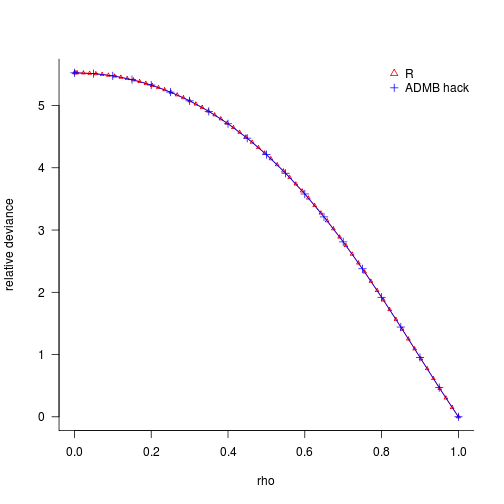## Junk

• have write_dat check vs. TPL file for order?
• is there a problem specifying an upper bound as something that's an init_number? ## write_dat(fn,list(dvar=dvar,nt=length(dvar)+1))